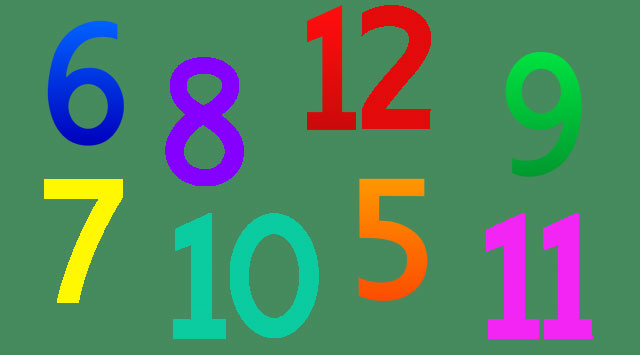# Cool Math Magic Tricks

Cool Math Magic Tricks | MathJokes.net
Hi everyone! Mathematics is one of the basic school subjects. Some people find exact sciences enlightening, others consider mathematic to be incredibly boring. And now, we are going to prove you that numbers can be fascinating. This is an amazing and incredible cool math magic trick with the numbers. No preparation needed and can be performed anywhere any time. Math magic about prediction number trick, is very effective but easy. I am loving this math tricks and I am sure you are gona love it too. Read and learn it.. then have fun fooling your friends.Math Magic Tricks

## Math Magic Tricks

Surprising Predictions
If you pause, it won’t work.
Here we go...
5 + 3 =
8
9 + 2 =
11
10 - 6 =
4
Quick !
Think of a number between 5 and 12
Got it?Cool Math Number Tricks

Now i'm going to guess which number you picked!
Here goes!
You picked...
.
.
.

7

***

## Math Number Tricks

• Think of a Number
• Multiply it by 2
• Divide by 2
• Subtract the number you started with
• The number is...
.
4
***

• Think of a Number
• Double it
• Subtract the number you first thought of
• Subtract it again
• The number is...
.
6
***

Explain:
Everybody started with different numbers, but ended up with the same number. Why?
The number you first thought of is always eventually subtracted.
Everyone has performed the same operations so you all end up with the same answer.

Example:
• Think of a number : n
• Double it : 2n
• Add 3 : 2n + 3
• Multiply by 4 : 8n + 12
• Subtract 12 : 8n
• Divide by 8 : n

***

• Think of a Number
• Subtract 3
• Subtract original number.
***

• Think of a Number
• Double it
• Subtract original number
• Subtract 1
• Subtract original number.
***

• Think of a Number
• Double it
• Subtract original number
• Subtract original number.
***

• Think of a Number
• Treble it
• Subtract original number
• Halve it
• Subtract original number
***

## Math Mind Tricks

Now i will guess your name!
What you are about to read is a fun, awesome math trick.
for this to work, you must follow the steps carefully!
The math is fairly simple, but if you need a calculator it's okay.
step 1
Have your starting number be the number of letters you have in your name
(e.g : Thomas = 6 letters)

step 2
If you have any of these letters; A, E, I, O, or U in your name, add 5.

step 3
if you are a boy, multiply your total by 2.
if you are a girl, multiply your total by 4.

step 4
if you are a boy, take away 4 from your total.
if you are a girl, take away 8 from your total.

step 5
if you are a boy, halve your total.
if you are a girl, quarter your total.

step 6
Now, substract the number of letters in your name from your total.

step 7
if you are a boy, you are done.
if you are a girl, add 5.

Now you should be left with a 1-digit or 2-digit number.
1 = Jack
2 = Anna
3 = Mr...
4 = Ryan
5 = Donna
6 = Amy
7 = Peter
8 = Mrs...
9 = Joseph
10 = Susan
11 = Bryan
12 = Jennifer
13 = Kelly
14 = Janet

3 or 8 = Mr/Mrs... Got you! April fools :)
***

This is a math magic tricks video from youtube. Watch this...

## Amazing Cool Math Tricks

Take the first 2 digits of your home phone number and add them to the last 2 digits of your phone number.
Multiply by 365.
Write the number on a new sheet of paper.
Fold the first page in half.
Now fold the second page.
Place them side by side.
Now pick up the two sheets - sheet one in your left hand and sheet two in your right hand.
Find a bin/drawer and place the sheets in it.